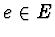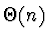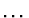CSE 5311 Fall 1999

Quiz #10

1.
(14 points). Let G = (V, E) be a flow network with source s and sink t in which each edgeis restricted to capacity c(e) = 1. Further suppose that a maximum flow for G has been computed and an edge is now removed from E. Describe how the maximum flow can be efficiently updated and give the run time of your algorithm.

2.
(6 points). Analyze the run time of the parallel code below. Also compute the speedup, assuming that the serial run time isand the number of processors p is >= n. In this algorithm assume that there are three arrays (A[1n], B[1n], and C[1k]) used, and each element i of array C is predefined to contain the number of elements from array A equal to i. Each element of arrays B and C are stored on a separate processor.

   ParallelAlg(A, B, C, k)
for each processor j, in parallel
C[j] = C[j] + C[j-1]
for each processor j, in parallel
B[C[A[j]]] = A[j]
C[A[j]] = C[A[j]] - 1


Is this parallel algorithm work efficient? Which sorting technique does this most closely resemble?